# Interval estimator

for the unknown true value of a scalar parameter of a probability distribution

An interval belonging to the set of admissible values of the parameters, with boundaries that are functions of the results of observations subject to the given distribution. Letbe a random variable taking values in a sample space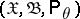,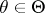,an interval on the real axis, where the true value ofis unknown. An interval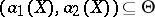with boundaries that are functions of the random variablebeing observed is called an interval estimator, or confidence interval, for; the numberis called the confidence coefficient of this confidence interval, andandare called the lower, respectively, upper, confidence bounds. The concept of an interval estimator has been generalized to the more general case when it is required to estimate some function, or some value of it, depending on a parameter.

Suppose that on a set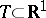a family of functions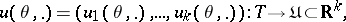has been given, and suppose that it is required to estimate the functioncorresponding to the unknown true value ofusing the realization of a random vector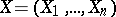taking values in the sample space,,. To eachcorresponds a set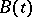, which is the image ofunder. By definition, a set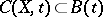is called a confidence set for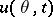if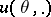at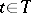has confidence probability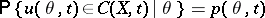and confidence coefficient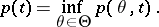The totality of all confidence sets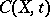forms in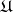the confidence region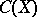forwith confidence probability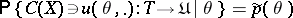and confidence coefficient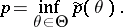Sets of the typeorare called interval estimators for one valueof a functionat a point and for the function, respectively.

There are several approaches to the construction of interval estimators for independent parameters of a distribution. The best known are the Bayesian approach, based on the Bayes theorem, Fisher's method, based on the fiducial distribution (for Fisher's method, see ), the Neyman method of confidence intervals (, , ), and the method proposed by L.N. Bol'shev .

How to Cite This Entry:
Interval estimator. M.S. Nikulin (originator), Encyclopedia of Mathematics. URL: http://encyclopediaofmath.org/index.php?title=Interval_estimator&oldid=17171
This text originally appeared in Encyclopedia of Mathematics - ISBN 1402006098